Home

## Abstract

Let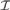${\cal I}$ be an ideal on ω. By cov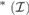${}_{}^{\rm{*}}({\cal I})$ we denote the least size of a family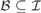${\cal B} \subseteq {\cal I}$ such that for every infinite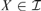$X \in {\cal I}$ there is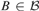$B \in {\cal B}$ for which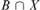$B\mathop \cap \nolimits X$ is infinite. We say that an AD family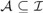${\cal A} \subseteq {\cal I}$ is a MAD family restricted to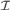${\cal I}$ if for every infinite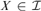$X \in {\cal I}$ there is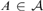$A \in {\cal A}$ such that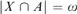$|X\mathop \cap \nolimits A| = \omega$ . Let a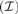$\left( {\cal I} \right)$ be the least size of an infinite MAD family restricted to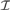${\cal I}$ . We prove that If$max$ {a,cov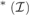${}_{}^{\rm{*}}({\cal I})\}$ then a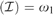$\left( {\cal I} \right) = {\omega _1}$ , and consequently, if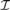${\cal I}$ is tall and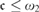$\le {\omega _2}$ then a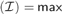$\left( {\cal I} \right) = max$ {a,cov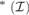${}_{}^{\rm{*}}({\cal I})\}$ . We use these results to prove that if c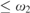$\le {\omega _2}$ then o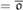$= \overline o$ and that as$= max$ {a,non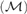$({\cal M})\}$ . We also analyze the problem whether it is consistent with the negation of CH that every AD family of size ω1 can be extended to a MAD family of size ω1.

• # Send article to Kindle

Note you can select to send to either the @free.kindle.com or @kindle.com variations. ‘@free.kindle.com’ emails are free but can only be sent to your device when it is connected to wi-fi. ‘@kindle.com’ emails can be delivered even when you are not connected to wi-fi, but note that service fees apply.

Find out more about the Kindle Personal Document Service.

Available formats
×

# Send article to Dropbox

To send this article to your Dropbox account, please select one or more formats and confirm that you agree to abide by our usage policies. If this is the first time you use this feature, you will be asked to authorise Cambridge Core to connect with your <service> account. Find out more about sending content to Dropbox.

Available formats
×

# Send article to Google Drive

To send this article to your Google Drive account, please select one or more formats and confirm that you agree to abide by our usage policies. If this is the first time you use this feature, you will be asked to authorise Cambridge Core to connect with your <service> account. Find out more about sending content to Google Drive.

Available formats
×

Hide All

## Metrics

### Full text viewsFull text views reflects the number of PDF downloads, PDFs sent to Google Drive, Dropbox and Kindle and HTML full text views.

Total number of HTML views: 0
Total number of PDF views: 0 *Loading metrics...

### Abstract viewsAbstract views reflect the number of visits to the article landing page.

Total abstract views: 0 *Loading metrics...

* Views captured on Cambridge Core between <date>. This data will be updated every 24 hours.

Usage data cannot currently be displayed.